Data Structure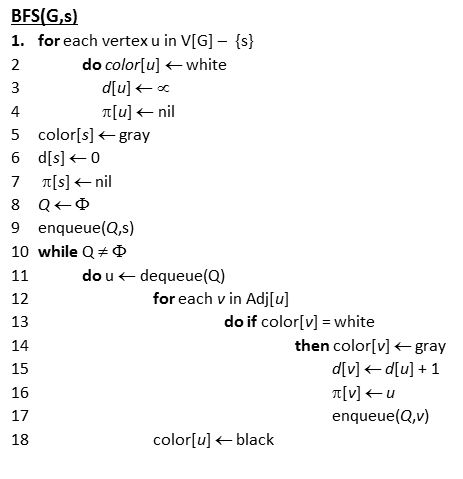• Q: a queue of discovered vertices
• color[v]: color of v
• d[v]: distance from s to v
• π[u]: predecessor of v
• white: undiscovered
• gray: discovered
• black: finished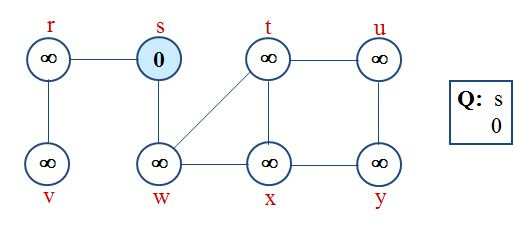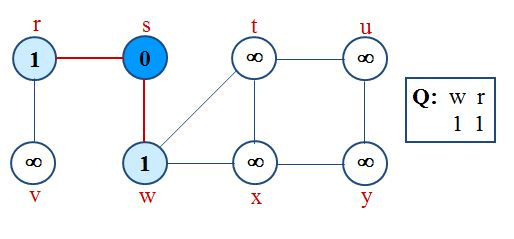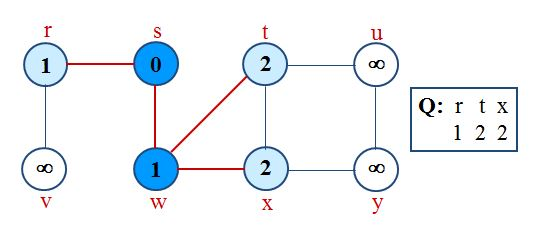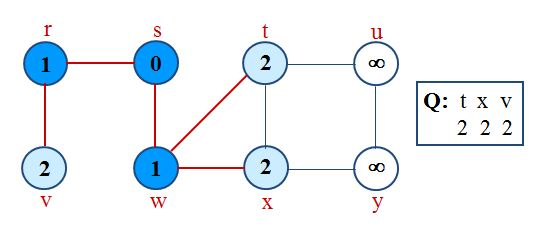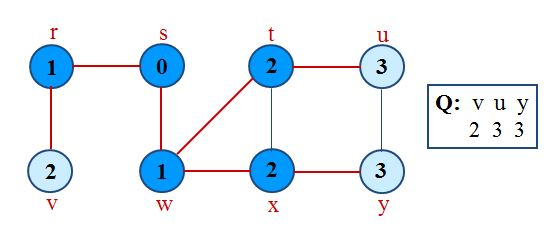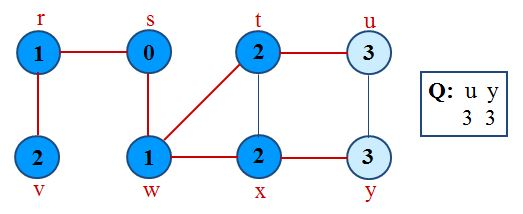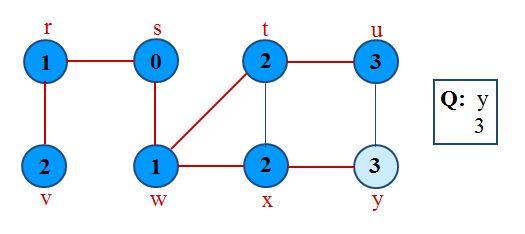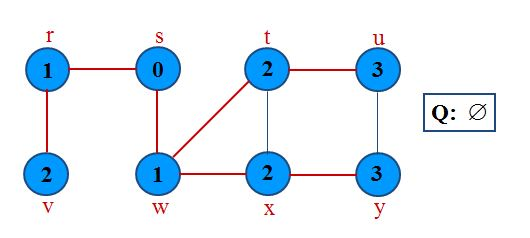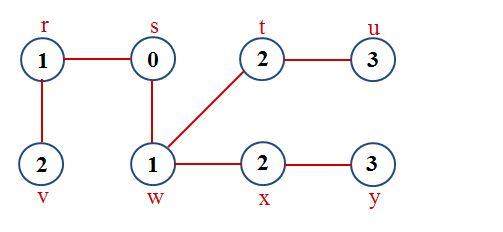Analysis of BFS

Initialization takes O(V).
Traversal Loop After initialization, each vertex is enqueued and dequeued at most once, and each operation takes O(1). So, total time for queuing is O(V).
The adjacency list of each vertex is scanned at most once. The sum of lengths of all adjacency lists is ?(E).

Summing up over all vertices => total running time of BFS is O(V+E), linear in the size of the adjacency list representation of graph.